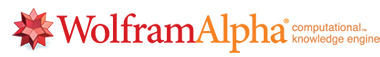# Search and find , solution and answer with Wolfram Alpha PART 3Wolfram|Alpha introduces a fundamentally new way to get knowledge and answers—
not by searching the web, but by doing dynamic computations based on a vast collection
of built-in data, algorithms, and methods.

PART 3 : Lets look at the mathematical searches and plotting

The following is not intended to be a handbook,
but only a listing of search examples

Calculus & Analysis

Integrals
compute an indefinite integral
integrate x^2 sin^3 x dx
compute a definite integral
integrate sin x dx from x=0 to pi
compute an improper integral
int sinx/x dx, x=0..infinity

Derivatives
calculate the derivative of a function
derivative of x^4 sin x
compute higher derivatives
second derivative of sin(2x)
compute partial derivatives
d/dx x^2 y^4, d/dy x^2 y^4

Limits
compute a limit
lim (sin x - x)/x^3 as x->0
limit (1+1/n)^n as n->infinity
compute a one-sided limit
lim x/|x| as x->0+

Sequences
analyze a sequence
sequence of Fibonacci numbers
compute a possible formula and continuation for a sequence
1, 4, 9, 16, 25, ...
solve a recurrence
g(n+1)=n^2+gSums
compute an indexed sum
sum j^2, j=1 to 100
compute an infinite sum
sum (3/4)^j, j=0..infinity

Products

compute an indexed product
product (k+2)/k, k=1..25
compute an infinite product
product (1-1/n^4), n=2 to infinity

Series Expansions

find a Taylor series expansion
taylor series sin x
specify the center point and the order of the expansion
series (sin x)/(x-pi) at x=pi to order 10

Coordinate Geometry

compute the area bounded by two curves
compute the area between y=|x| and y=x^2-6
locate inflection points of a function
inflection points of x+sin(x)

Differential Equations

solve a linear ordinary differential equation
y'' + y = 0
specify initial values
y'' + y = 0, y(0)=2, y'(0)=1
solve a nonlinear equation
f'(t) = f(t)^2 + 1

Complex Analysis

compute properties of a function of a complex variable (use the variable z)
e^z
compute the residue of a function at a point
residue of 1/(z^2+4)^2 at z=2i

Vector Analysis
compute the gradient of a function
calculate alternate forms of a vector analysis expression
curl (curl F)

Integral Transforms

compute a Fourier transform
Fourier transform exp(-x^2)
compute a Laplace transform
LT e^t sin 2t

Domain & Range
compute the domain of a function
domain of f(x) = x/(x^2-1)
compute the range of a function
range of e^(-x^2)

There are no comments to display.

Author
kak111